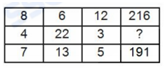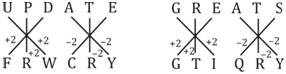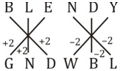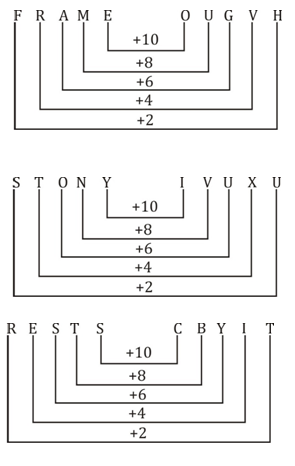# SSC Reasoning Ability Practice Questions (Day-82)

Dear Aspirants, The SSC Exams are going on at national level. Millions of candidates have eagerly prepared for the ongoing SSC exams such as CGL, CHSL, MTS, CPO, JE etc. SSC exams are usually conducted by the Staff Selection Commission to recruit the eligible candidates for various Central government departments. Mostly the selection process for all the SSC exams consists of the single or double phase of written examination based on the examination. Due to that, the written exam consisted of a certain syllabus and exam pattern. By following those regulations, the written exam has been conducted through an online mode. Based on the latest updated exam pattern and syllabus, here we have drafted the Reasoning Ability questions on a daily basis. So aspirants start your practice session with us and mould your skill in a perfect shape.

Start Quiz

1) 6 boys P, Q, R, S, T and U are standing in a row facing north. P and Q cannot be either at 1st or 2nd place. R and S will always be together and R mustbe at one of the ends. R doesn’t have S to his right. Who is standing on the west end?

(a) S

(b) S or U

(c) T

(d) T or U

Direction (2-3): In the following question, select the word which cannot be formed using the letters of the given word.

2) BLENDING

(a) BLEND

(b) BALD

(c) LEND

(d) BINGE

(a) NATIONAL

(b) TRAIN

(c) LAND

(d) RATION

4) In a certain code language, “UPDATE” is written as “FRWCRY” and “GREATS” is written as “GTIQRY”. How is “BLENDY” written in that codelanguage?

(a) DMGWBL

(b) CJZAFP

(c) ZJCPFA

(d) GNDWBL

5) In a certain code language,”FRAME” is written as “OUGVH” and “STONY” is written as “IVUXU”. How is “RESTS” written in that code language?

(a) CBYIT

(b) ABDHS

(c) EAZHS

(d) EAZIT

6) If “+” denotes “multiplied by”, “–” denotes “added to”, “×” denotes “divided by” and “÷” denotes “subtracted from”, then which of the following equationis true?

(a) 30 ÷ 8 × 4 – 6 + 1 = 32

(b) 8 + 5 – 20 × 4 ÷ 6 = 41

(c) 12 – 12 ÷ 6 × 6 + 3 = 21

(d) 6 + 7 × 3 – 4 ÷ 6 = 26

7) In the following question, by using which mathematical operators will the expression become correct?

35 ?5 ?10 ?15 ?4

(a) ÷, +, = and ×

(b) ×, ÷, > and ×

(c) ÷, ×, > and ×

(d) ÷, ×, < and +

8) If 34 × 15 = 495 and 43 × 12 = 504, then 98 × 17 = ?

(a) 1649

(b) 1683

(c) 1763

(d) 1751

9) If 26 (52) 8 and 48 (192) 16, then what is the value of ‘A’ in A (175) 14?

(a) 50

(b) 25

(c) 35

(d) 40

Direction (10): In the following question, select the number which can be placed at the sign of question mark (?) from the given alternatives.

10)(a) 152

(b) 184

(c) 166

(d) 162

T             U      P      Q       S      R

or

U      T      Q      P       S      R

T or U stands on the west end.

‘BALD’ ; There is no ‘A’ in the given word.

‘NATIONAL’ ; There is no double ‘N’ in the given word.12 – 12 ÷ 6 × 6 + 3 = 21

⇒ 12 + 12 – 6 ÷ 6 × 3 = 21

⇒ 12 + 12 – 3 = 21

⇒ 24 – 3 = 21

⇒ 21 = 21

35 ÷ 5 × 10 > 15 × 4

⇒ 7 × 10 > 60

⇒ 70 > 60

(34 × 15) – 15 = 495

(43 × 12) – 12 = 504

(98 × 17) – 17 = 1649

26/2 × 8/2 = 52

48/2 × 16/2 = 192

50/2 × 14/2 = 175

6 × (8 + 12) + 8 × 12 = 120 + 96 = 216

13 × (7 + 5) + 7 × 5 = 156 + 35 = 191

22 × (4 + 3) + 4 × 3 = 154 + 12 = 166

 Check Here to View SSC CGL / CHSL 2021 Reasoning Ability Questions Day – 81 Day – 80 Day – 79